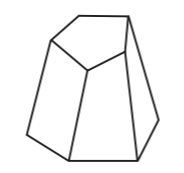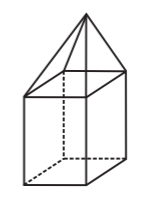# Class 8 NCERT Solutions – Chapter 10 Visualising Solid Shapes – Exercise 10.3

• Last Updated : 24 Nov, 2020

### (i) 3 triangles? (ii) 4 triangles? (iii) a square and four triangles?

Solution:

(i) 3 triangles: No, because polyhedron must have minimum 4 faces i.e all edges should meet at vertices.

Attention reader! All those who say programming isn't for kids, just haven't met the right mentors yet. Join the  Demo Class for First Step to Coding Coursespecifically designed for students of class 8 to 12.

The students will get to learn more about the world of programming in these free classes which will definitely help them in making a wise career choice in the future.

(ii) 4 triangles: Yes, as all the edges are meeting at the vertices and has four triangular faces.

(iii) a square and four triangles: Yes, because all the eight edges meet at the vertices having a square face and four triangular faces.

### Question 2: Is it possible to have a polyhedron with any given number of faces? (Hint: Think of a pyramid).

Solution:

Yes, It is possible to have a polyhedron with any given faces only if the number of faces are greater than or equal to four.

### Question 3: Which are prisms among the following?Solution:

Prisms among the given images are

(ii) Unsharpened pencil

(iv) A box.

### Question 4: (i) How are prisms and cylinders alike? (ii) How are pyramids and cones alike?

Solution:

(i) If the number of sides in a prism are increased to certain extent, then the prism will take the shape of cylinder i.e. a prism with a circular base.

(ii) If the number of sides of the pyramid is increased to same extent, then the pyramid becomes a cone i.e. a pyramid with a circular base.

### Question 5: Is a square prism same as a cube? Explain.

Solution:

Yes, a square prism can be same as a cube, but if the height of the prism is greater than It may be cuboid.

### Question 6: Verify Euler’s formula for these solids.

(i)(ii)Solution:

(i) No. of Faces (F) = 7

No. of Vertices (V) = 10

No. of Edges (E) = 15

By Using Euler’s formula: F + V – E = 2 and Substituting the values, we get

⇒ 7 + 10 – 15 = 2

⇒ 2 = 2

Therefore, Euler’s formula is verified.

(ii) No. of Faces (F) = 9

No. of Vertices (V) = 9

No. of Edges (E) = 16

By Using Euler’s formula: F + V – E = 2 and Substituting the values, we get

⇒ 9 + 9 – 16 = 2

⇒ 2 = 2

Therefore, Euler’s formula is verified.

### Question 7: Using Euler’s formula find the unknown.

Solution:

(i)

No. of Faces (F) = F

No. of Vertices (V) = 6

No. of Edges (E) = 12

By Using Euler’s formula: F + V – E = 2 and Substituting the values, we get

⇒ F + 6 – 12 = 2

⇒ F = 2 + 6

⇒ F = 8

Therefore, No. of Faces (F) = 8

(ii)

No. of Faces (F) = 5

No. of Vertices (V) = V

No. of Edges (E) = 9

By Using Euler’s formula: F + V – E = 2 and Substituting the values, we get

⇒ 5 + V – 9 = 2

⇒ E = 2 + 4

⇒ E = 6

Therefore, No. of Vertices (V) = 6

(iii)

No. of Faces (F) = 20

No. of Vertices (V) = 12

No. of Edges (E) = E

By Using Euler’s formula: F + V – E = 2 and Substituting the values, we get

⇒ 20 + 12 – E = 2

⇒ E = 32 – 2

⇒ E = 30

Therefore, No. of Edges (E) = 30

### Question 8: Can a polyhedron have 10 faces, 20 edges and 15 vertices?

Solution:

Since every polyhedron satisfies Euler’s formula, therefore checking if polyhedron can have 10 faces, 20 edges and 15 vertices.

No. of Faces (F) = 10

No. of Vertices (V) = 15

No. of Edges (E) = 20

By Using Euler’s formula: F + V – E = 2 and Substituting the values, we get

⇒ 10 + 15 – 20 = 2

⇒ -5 = 2

As Euler’s formula is not satisfied, therefore polyhedron cannot have 10 faces, 20 edges and 15 vertices.

My Personal Notes arrow_drop_up